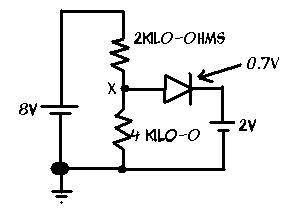# Simple Diode Circuit

I have an interesting question about this circuit... if I were to calculate the voltage from point X to ground... essentially all it is, is voltage across the 4 kilo-ohm resistor because if it went the route of the diode, it would lose an additional 2.7 volts.. so its going to take the better path.. right? Thats how it works doesn't it?

so... we have... $$\frac{4k}{2k+4k} * 8 Volts = 5.7 V$$

Now... lets say we reversed the diode... wouldn't then.. we push 1.7V towards point X right? Now... does that 1.7 volts add up with the 5.7V, does the 5.7 volts push against the 1.7V. The voltage I know is still 5.7 volts even if the diode was reversed... but is that because the voltage won't go that way due to there being more across the 4 kilo-ohm resistor?

Picture of circuit:Last edited:

I got 5.3333333333 Volts using your method, and if the diode was forward biased, why wouldn't point X be 2.7 Volts? I think this will cause some current to oppose the 2V source

NoTime
Homework Helper
For the original circuit the Diode/Battery is the functional equivilent of a zener diode.

For the case where the diode is reversed.
What is the basic function of a diode?
What happens when a diode is reverse biased?

oops I ment to write 5.3 not 5.7 for everything

When a diode is reversed current doesn't go through it.

But what I'm wondering is why you don't add the resultant voltage with the 5.3V

Last edited:
Yes, the voltage at X is 5.3V. Subtract 0.7V from 5.3V and it's still more than 2V. So the 8V battery is charging the 2V battery through the diode, which if forward-biased.